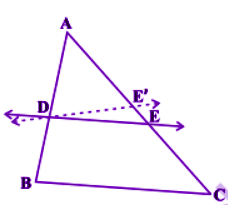# State and prove converse of BPT (basic proportionality theorem)

Statement of basic proportionality theorem (BPT)

According to this theorem, if a line divides any two sides of a triangle in the same ratio, then the line is parallel to the third side.

Proof:Suppose a line DE, intersects the two sides of a triangle AB and AC at D and E, such that;

Assume DE is not parallel to BC. Now, draw a line DE’ parallel to BC.

Hence, by similar triangles,

From eq. 1 and 2, we get;

AE/EC = AE’/E’C

Adding 1 on both the sides;

AE/EC + 1 = AE’/E’C +1

(AE +EC)/EC = (AE’+E’C)/E’C

AC/EC = AC/E’C

So, EC = E’C

This is possible only when E and E’ coincides.

But, DE’//BC

Therefore, DE//BC.

Hence, proved.